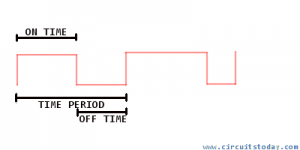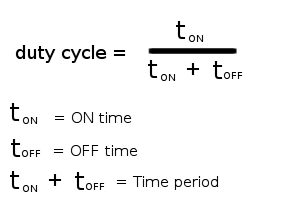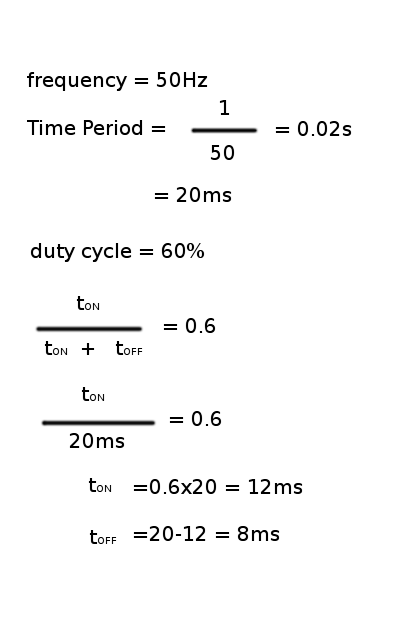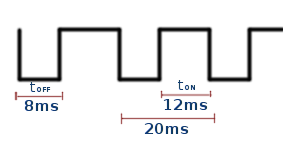## Audio

[Audio][slideshow]

The good definition of Pulse Width Modulation (PWM) is in the name itself. It means modulating/varying the width of the pulse (Not the frequency). To best understand what PWM is, let us first see some basic terminologies.

Microcontrollers are intelligent digital components which live on binary signals. Best representation of a binary signal is a square wave. The following diagram explains the basic terminologies associated with a square wave signal.As the shown in the above diagram, the important thing to note in a PWM signal is that the time-period and hence the frequency is always fixed. Only the ON time and OFF time of the pulse (duty cycle) varies. By this technique, we can modulate the given voltage.The one difference between a square wave signal and PWM signal is that the square wave signal has same ON and OFF time (50% duty cycle), whereas a PWM signal has a variable duty cycle. The square wave can be taken as a special case of the PWM signal which has 50% duty cycle (ON time = OFF time).

For example,
Given a supply voltage of 50V, and if the load needs 40 volts, the good way to generate 40v out of 50v is to implement a step-down chopper (More on choppers). The chopper needs a firing signal which must be a PWM signal to turn ON and OFF the thyristor. This PWM signal can easily generated by a microcontroller having timer units. The requirements for the PWM signal to generate a 40v using a thyristor from 50v supply is, ON time = 400ms, OFF time = 100ms (given the period of the PWM signal is 500ms). In common words this can be easily explained in the following way: Basically, a thyristor is operated as a switch. In a step-down chopper, the load gets the current from the source via the thyristor. When the thyristor is in OFF condition, the load is not connect to the source and when thyristor is in ON condition, the load is connected to the source. This work of switching ON and OFF a thyristor is done by the PWM signal. The percentage of time period the PWM signal is high/ON is called the duty cycle of the signal. If the duty cycle is 100% then the wave becomes a constant DC. So, the duty cycle can be calculated using the following formula:By using the above formula, we can calculate the tON for desired voltage we want from the total source voltage. By multiplying the duty cycle with 100, we can represent it in percentage. Thus the percentage of duty cycle is directly proportional to the amount of the voltage taken from the source. In the above example provided, if we want, 40 volts from a 50volts source, this can be accomplished by a step down chopper of duty cycle 80%. Since 80% of 50 is 40.

### At a frequency 50Hz design a PWM wave with duty cycle 60%.The resulting PWM wave will be :One of the best example application of pulse width modulation is to use PWM for motor speed control.

This technique of modifying the width of the pulse to get the desired duty cycle is called “Pulse width modulation”.

[Lights][grids]